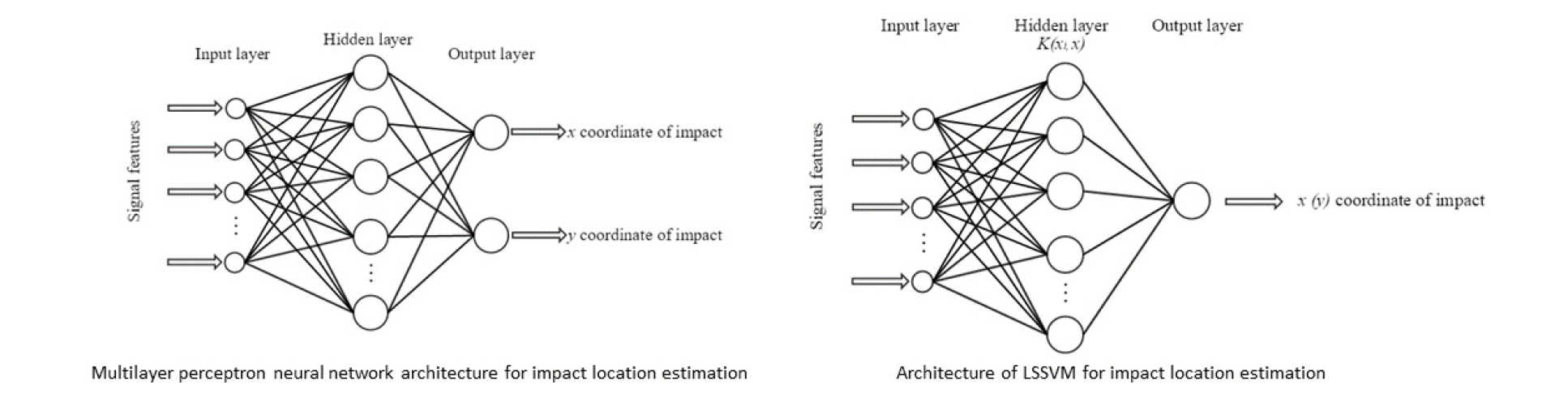In our research, machine learning algorithms are used for developing response surfaces or  meta-models for robust and reliability based optimization and for passive sensing using supervised learning. Artificial Neural Network (ANN) as well as Support Vector Machines (SVM) are used for developing non-linear relationships between a set of input and output data which can be used for topological optimization, robust and  reliability based optimization as well as pattern recognition and classifications. Different ANN architectures with different activation functions are used.

ANN is a machine learning algorithm that uses a set of training data to build a non-linear function relating a set of input data to output data. If there are too many parameters in the model compared to the number of training patterns, there might be the problem of overfitting. One way to ensure generalisation is to use enough data for training each network weight or to use an early stopping regularization.

Another class of neural networks is Support Vector Machine (SVM) which is suitable for modelling non-linear systems. Unlike ANN, the architecture of a SVM model is determined automatically by solving the convex optimization problem.  A modified model called Least Square SVM (LS-SVM) simpliﬁes the original SVM by adopting equality constraints in stead of inequality constrains.